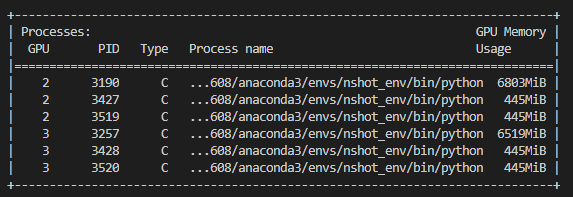# DistributedDataParallel causes Dataloader workers to utilize GPU memory

Hi everyone, I’m dealing with a very bizarre problem that I’m not sure how to solve.

I’m finding that whenever I use DistributedDataParallel where each process creates a Dataloader with num_workers > 0 set, I see that in `nvidia-smi` that several worker processes are spawned that are each utilizing about 500 MiB.

Whenever I don’t use DistributedDataParallel, the only process I see utilizing GPU memory is the main process (no worker processes are shown). I am extremely confident that there is no code in the dataset get methods or collate functions that moves the tensors to GPU memory.

This issue can persist in two different ways. If I wrap my model in DDP first, I notice these processes claim GPU memory when the dataloader is being created. Vice-versa, if I make my dataloader first, I notice these processes claim GPU memory when the model is being wrapped in DDP.

I don’t believe this is expected behavior, I don’t understand why the worker processes would even want GPU memory when they should just be handling fetching the data into RAM. Any guidance on this problem will be appreciated.

Continue discussion from Should I use 'spawn' method to start multi-processing?

Could you please show the output of `nvdia-smi`? Do you see any process id that appears on both GPUs?

A min repro code will be helpful.

I’m in the process of reproducing the issue in a separate code-base. In an initial isolated test, I’m actually not seeing this issue, but I am seeing it the code-base I’m working on… I’m not able to share that code-base, but I’m going to continue trying to replicate the problem.

For now, here’s a screenshot of what I’m seeing:3190 and 3257 both look normal to me. The other processes are workers that are spawn that start to utilize GPU memory somehow. I’m not very familiar with the inner-workings of CUDA, but could it be that the worker processes are running into code that thinks it needs a CUDA context? Thus allocating room for it on each worker process?

I am not sure, but the size (445MB) does look like CUDA context. cc DataLoader experts @VitalyFedyunin @SimonW @vincentqb

Looks like CUDA context to me. So probably your dataset code somehow uses CUDA. Also, if you are using `spawn` (default for windows and mac), make sure to wrap all code that may initialize CUDA in `if __name__ == '__main__'`

I have narrowed it down! I found that the dataset object is being assigned a class method from a nn.Module class. Thus the solution is to not assign a module’s class method to the dataset. Here is a code snippet that reproduces the problem. NOTE that this problem does not occur if DDP is not used.

I would like to have a high level understanding of this issue. Why would assigning a class method to the dataset cause the worker processes to have CUDA context? Additionally, why is this only occurring when using the DDP module?

``````import torch.multiprocessing as mp
import torch.distributed as dist
import torch.nn as nn
import torchvision
import os

from torch.nn.parallel import DistributedDataParallel as DDP
from torchvision.datasets import MNIST
import time

class SimpleModel(nn.Module):
def __init__(self):
super().__init__()
self.linear1 = nn.Linear(10, 10)

def forward(self, x):
return x

def preprocess(self, batch):
return batch

def main():

mnist_dataset = MNIST(
transform=torchvision.transforms.ToTensor()
)
model = SimpleModel()
is_parallel = True

if is_parallel:
mp.spawn(train_wrapper, nprocs=2, join=True,
args=(model, mnist_dataset))
else:
train(model, mnist_dataset, False, 'cuda:1')

def train_wrapper(rank, model, train_data):
os.environ['MASTER_PORT'] = '12345'

devices = ['cuda:1', 'cuda:2']

dist.init_process_group(backend='nccl', rank=rank, world_size=len(devices))
train(model, train_data, True, devices[rank])
dist.destroy_process_group()

def train(model, train_data, is_parallel, device):

# NOTE: THIS IS THE PROBLEM LINE
# If you comment this line out, the issue no longer persists
train_data.preprocess = model.preprocess

train_data,
num_workers=4,
batch_size=16
)

model = model.to(device)

if is_parallel:
model = DDP(model, device_ids=[device])